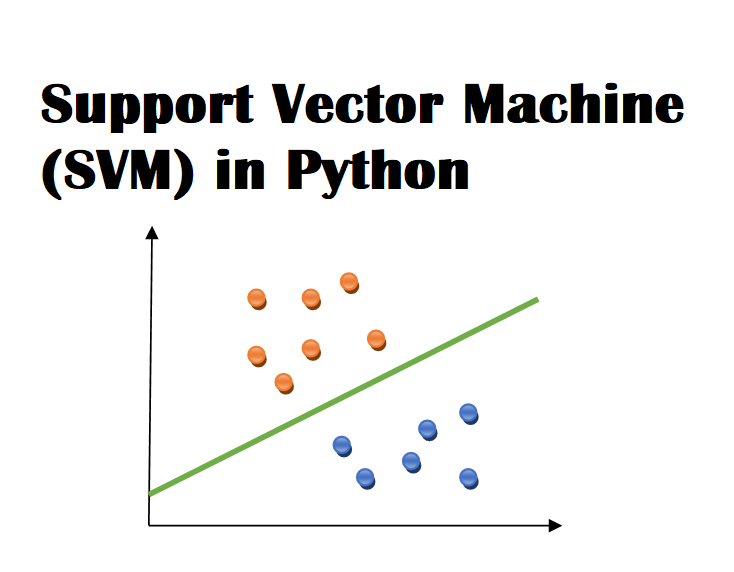# Implementing Support Vector Machine (SVM) in Python

Machine Learning is the most famous procedure of foreseeing the future or arranging data to help individuals in settling on essential choices.

The algorithms are trained over models through which they gain information from past encounters so as to make forecasts about what’s to come.

There are three types of Machine learning i.e. supervised learning, unsupervised learning and reinforcement learning.

In this article, I want to acquaint you with a predominant machine learning technique known as Support Vector Machine (SVM).

Before we start it formally, it is essential to know about supervised machine learning: –

## Supervised Machine Learning

In supervised machine learning, a labeled dataset is used. You must have input variables (X) and output variables (Y) then you apply an appropriate algorithm to find the mapping function from input to output.

Y = f(X)

Supervised machine learning can be categorized into the following:-

1. Classification – where the output variable is a category like black or white, plus or minus. Naïve Bayes (NB), Support Vector Machine (SVM) and Decision Tree (DT) are the most trendy supervised machine learning algorithms.
2. Regression – where the output variable is a real value like weight, dollars, etc. Linear regression is used for regression problems.

## Support Vector Machine

Support Vector Machine (SVM) belongs to a supervised machine learning algorithm which is mostly used for data classification and regression analysis.

We can perform linear and non-linear classification with the help of Support Vector Machine.

SVM Classifier splits the data into two classes using a hyperplane which is basically a line that divides a plane into two parts.

## Applications of Support Vector Machine in Real Life

As you already know Support Vector Machine (SVM) based on supervised machine learning algorithms, so, its fundamental aspire to classify the concealed data.

It is most popular due to its memory efficiency, high dimensionality and versatility. There are several applications of SVM in real life some of them are mentioned here.

• Face detection
• Image classification
• Reorganization of Handwriting
• Geo and environmental sciences
• Bioinformatics
• Text categorization
• Protein fold and remote homology detection
• Generalized predictive control

## Examples of SVM Kernels

1. Polynomial kernel – it is mostly used in image processing.
2. Linear Splines kernel in one-dimension – it is used in text categorization and is helpful in dealing with large spare data vectors.
3. Gaussian Kernel – it is used when there is no preceding information about the data.
4. Gaussian Radial Basis Function (RBF) – It is commonly used where there is no previous knowledge about the data.
5. Hyperbolic Tangent Kernel – it is used in neural networks.
6. Bessel Function of the First kind Kernel – it is used to eliminate the cross term in mathematical functions.
7. Sigmoid Kernel – it can be utilized as the alternative for neural networks.
8. ANOVA Radial Basis Kernel – it is mostly used in regression problems.

## Support Vector Machine (SVM) implementation in Python:

Now, let’s start coding in python, first, we import the important libraries such as pandas, numpy, mathplotlib, and sklearn.

``````import pandas as pd
import numpy as np
from sklearn import linear_model
import matplotlib.pyplot as plt``````

Load the dataset:

Now we load the dataset i.e. apples_and_oranges.csv which is already placed in the same folder where svm.ipynb file saved and also check the dataset what is inside the file. See this figure.

``````df = pd.read_csv('apples_and_oranges.csv')
df``````

We can also represent this data frame as a scatter plot.

``````plt.xlabel('weight')
plt.ylabel('size')
plt.scatter(df['weight'], df['weight'],color="green",marker='+', linewidth='5')
plt.scatter(df['size'], df['size'],color="blue",marker='.' , linewidth='5')``````

Split the dataset of Apples and Oranges into training and test samples with a ratio of 80% & 20%.

``````from sklearn.model_selection import train_test_split
train_set, test_set = train_test_split(df, test_size=0.2)``````

Now we classify the predictors and target.

``````x_train = train_set.iloc[:,0:2].values
y_train = train_set.iloc[:,2].values
x_test = test_set.iloc[:,0:2].values
y_test = test_set.iloc[:,2].values``````

We can also check the length of train_set and test_set by using this code

When we initialize the Support Vector Machine (SVM) and fitting the training data, we obtain.

``````from sklearn.svm import SVC
model = SVC(kernel='rbf', random_state = 1)
model.fit(x_train, y_train)``````

Now, we will check the accuracy of our model.

``model.score(x_test, y_test)``

Wao… our model worked perfectly as it provides 100% accuracy but this may not happen all the time especially in the case where a large number of features are involved.

Now, we will predict the class of a fruit whose weight is 55 and size is 4.

``model.predict([[55,4]])``

Another check to predict the class of a fruit whose weight is 60 and size is 5.50.

``model.predict([[60,5.50]])``

Hence, it is clear from above that the Support Vector Machine (SVM) is an elegant and dominant algorithm.

We can also use another kernel i.e. linear and check the model score like this.

``````model_linear_kernal = SVC(kernel='linear')
model_linear_kernal.fit(x_train, y_train)``````
``model_linear_kernal.score(x_test, y_test)``

Problem Statement No.1:

Train a Support Vector Machine (SVM) Classifier by using any suitable dataset and then find out the accuracy of your model by utilizing rbf and linear kernels.

You can download dataset here.

### 3 thoughts on “Implementing Support Vector Machine (SVM) in Python”

1.I like the valuable information you supply in your articles. I’ll bookmark your blog and check once more here regularly. I’m fairly certain I will be informed lots of new stuff right here! Good luck for the next!

2.Like!! I blog frequently and I really thank you for your content. The article has truly peaked my interest.

3.Greate article. Keep posting such kind of info on your site.
Im really impressed by your site.
Hey there, You have done a fantastic job.
I will definitely digg it and individually recommend
to my friends. I am sure they’ll be benefited from this website.